ISEE Middle Level Math : How to find percentage

Example Questions

Example Question #21 : Percentage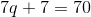What is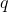?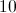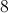Explanation:

First,subtract 7 from each side: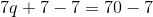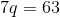Then, divide each side by 7: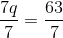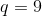Example Question #22 : Percentage

Berta went shopping for a bathroom curtain. How much did she pay for the curtain if it wasoff its original price of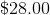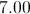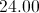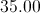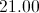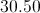Explanation:

First, multiply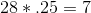.

Then, subtract that amount from the original price.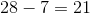Answer: Berta paid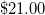for the curtain.

Example Question #21 : How To Find Percentage

Oliver pays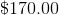for a skateboard. What is the discount percentage he receives if the skateboard originally costs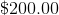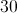Explanation:

Divide.

Subtract that amount fromto find the discount percentage Oliver received: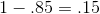Answer: Oliver received adiscount on the skateboard.

Example Question #21 : How To Find Percentage

A computer is on sale for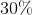off its original price of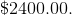What is the sales price of the computer?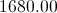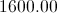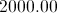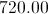Explanation:

First, multiply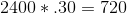Then, subtract that amount from the original price: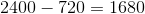Answer: The sales price is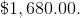A painting is 37% off of its original price of $200.00. What is the sale price of the painting? Possible Answers: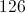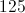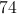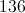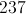Correct answer:Explanation: First, multiply: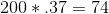The, subtract that amount from 200: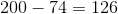Example Question #26 : Percentage A guitar is on sale for 15% off of its original price of$350.00. What is the sales price of the guitar?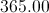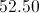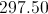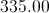Explanation:

First, multiply: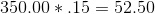Then, subtract that value from the original amount: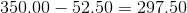Example Question #22 : How To Find Percentage

85 is what percent of 400?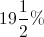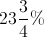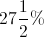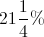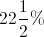Explanation:

Set up the proportion statement, whereis our answer, and solve: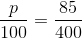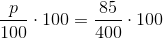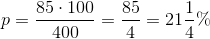Example Question #21 : How To Find Percentage

In a class of 120 students, 108 of them decide to attend a field trip. What percent of the students do not attend the field trip?

10%

12%

90%

88%

10%

Explanation:

If 108 students attend the field trip, 12 students do not.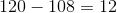To find the percent of students not attending the field trip, divide the number of students not attending by the total number of students, and then multiply that result by 100%.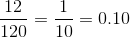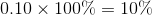Example Question #29 : Percentage

Below is the list of candidates for Student Council president, along with the number of votes each won: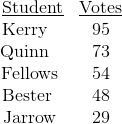What percent of the students voted for neither Kerry nor Quinn (nearest tenth)?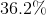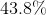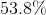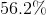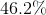Explanation: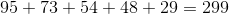students total voted. Out of those,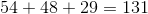voted for a candidate other than Kerry or Quinn.  To convert this to a percent, use this proportion and solve for: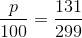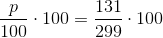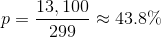Example Question #30 : Percentage

A $200.00 cell phone is on sale for 15% off. What is the sales price of the cell phone? Possible Answers: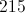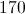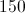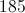Correct answer:Explanation: First multiply: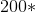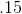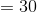Then, subtract that amount from the original price: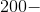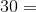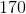Answer: The cell phone costs$170.00.